Science, Maths & Technology

### Become an OU studentEveryday maths 1

Start this free course now. Just create an account and sign in. Enrol and complete the course for a free statement of participation or digital badge if available.

# Session 4: Shape and space

## 1 Around the edge

When might you need to work out how far it is around a flat shape?

You will need to know how far it is around the edge of a shape when you want to put a border around something, such as a wallpaper border around a room, or a brick wall around a patio. You might have thought of different examples.

The distance around any shape is called the perimeter. You can work out the perimeter by adding up all of the sides. The sides are measured in units of length or distance, such as centimetres, metres or kilometres.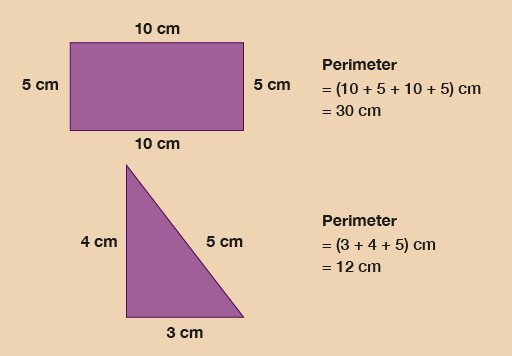Figure _unit5.1.1 Figure 1 Looking at perimeters

### Case study _unit5.1.1 Example: A length of ribbon

Have a look at the figure below to work out how much decorative ribbon you need to go around each shape.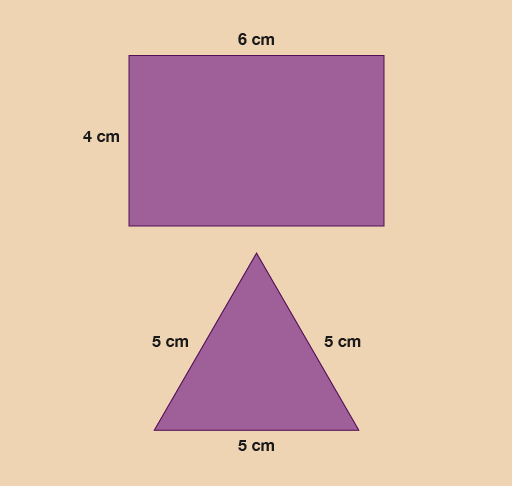Figure _unit5.1.2 Figure 2 Calculating the length of ribbon

#### Method

You need to measure all the sides and add them together.

##### Box _unit5.1.1

Hint: Opposite sides of a rectangle are the same length.

The sides of the rectangular box are:

##### Extract _unit5.1.1

6 + 6 + 4 + 4 = 20 cm

You will need 20 cm of ribbon.

The sides of the triangular box are:

##### Extract _unit5.1.2

5 + 5 + 5 = 15 cm

You will need 15 cm of ribbon.

### Case study _unit5.1.2 Example: A quicker calculation

So far when you have been working with perimeter you have added up all four sides, however there is a quicker way of calculating the perimeter. You may have recognised that all rectangles have two equal short sides and two equal long sides. Therefore you can then work out the perimeter by using each number twice.

##### Extract _unit5.1.3

(2 × long side) + (2 × short side) = perimeter

The long side is the length. The short side is the width.

(A square is a type of rectangle where all four sides are the same length. So to find out the perimeter of a square, the you need to multiply the length of one side by 4.)

How many metres of lawn edging do you need to go around this lawn?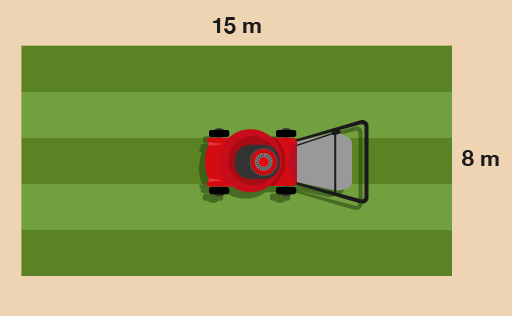Figure _unit5.1.3 Figure 3 A lawn

#### Method

You need to work out twice the width, plus twice the length:

##### Extract _unit5.1.4

(2 × 15) + (2 × 8)

Once you’ve worked these out, it makes the answer to the question easier to get:

##### Extract _unit5.1.5

(2 × 15) + (2 × 8) = 30 + 16 = 46 m

Now try the following activity. Remember to check your answers once you have completed the questions.

### Activity _unit5.1.1 Activity 2: Finding the perimeter

1. You need to hang bunting around the tennis courts for the local championships. How much bunting do you need?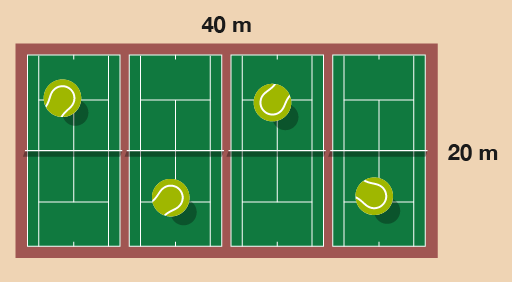Figure _unit5.1.4 Figure 4 Four tennis courts
1. How much tape does the police officer need to close off this crime scene?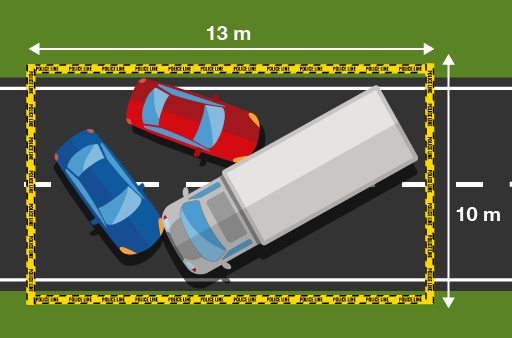Figure _unit5.1.5 Figure 5 A crime scene